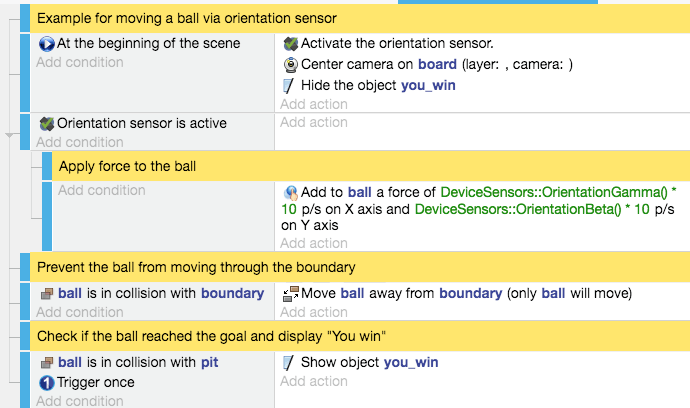# Device sensors

GDevelop has the ability to access the sensors that can be found on most mobile devices.

## Orientation sensor

The orientation sensor combines the gyroscope, accelerometer and compass in one sensor. This allows you to control game objects by slightly rotating the mobile device in your hands. You can access the location data via the "alpha", "beta" and "gamma" values which are explained in the next section.

### Axes explained

#### AlphaThe "alpha" value corresponds to the axis which points to the sky when your phone is lying on a table with its display pointing upwards. You can easily create a compass using the "alpha" rotation value.

Range: 0 to 360 degrees

#### BetaThe "beta" axis runs through the narrow edge of the phone.

Range: -180 to 180 degrees

#### GammaThe "gamma" axis runs through the longer edge of the phone.

Range: -90 to 90 degrees

### Conditions

Sensor Active

This condition checks whether the sensor has been activated yet and is delivering location data.

Orientation Alpha

This condition lets you compare the value of alpha to a number or variable.

Orientation Beta

This condition lets you compare the value of beta to a number or variable.

Orientation Gamma

This condition lets you compare the value of gamma to a number or variable.

### Actions

Activate Orientation Sensor

This action activates the orientation sensor so that it begins to deliver alpha, beta and gamma values. Turn this off when you no longer need the sensor to save some processing time and phone battery.

Deactivate Orientation Sensor

This action deactivates the orientation sensor so that it stops delivering alpha, beta and gamma values.

### Expressions

Is Absolute

This value indicates that the orientation data is in absolute values. In reference to the Earth's coordinates (value 1) or using an arbitrary frame, is determined by the device (value 0).

Alpha Value

The "alpha" rotation.

Beta Value

The "beta" rotation.

Gamma Value

The "gamma" rotation.

## Motion sensor

In contrast to the orientation sensor which returns absolute values, the motion sensor delivers relative ones. This sensor only returns values while the device is moving. If you hold it still, no matter its orientation, the sensor will return 0. You can access the motion data along an axis via the "x", "y", "z" values and the rotation data around an axis via "alpha", "beta" and "gamma" which are explained in the next section.

Note

The motion sensor rotation values (alpha, beta, gamma) are currently badly supported on mobile devices. It would currently be better to calculate the value from the orientation data

### Axes explained

#### Acceleration XThis value shows the acceleration along the x-axis. If you move your device along the longer edge, the x value will increase.

#### Acceleration YThis value shows the acceleration along the y-axis. If you move your device along the smaller edge, the y value will increase.

#### Acceleration ZThis value shows the acceleration along the z-axis. If you move your device up or down, the z value will increase.

#### Rotation alphaThis value shows the acceleration around the z-axis. If you turn your device with the display pointing up, the alpha value will increase.

#### Rotation betaThis value shows the acceleration around the x-axis. If you hold your device in landscape mode with a hand on each side, you can turn your device around the x-axis and the beta value will increase.

#### Rotation gammaThis value shows the acceleration around the y-axis. If you hold your device in portrait mode with a hand on each side, you can turn your device around the y-axis and the beta value will increase.

### Conditions

Sensor Active

This condition checks whether the sensor has been activated yet and is delivering location data.

Acceleration X

This condition lets you compare the value of x-acceleration to a number or variable. (m/s²)

Acceleration Y

This condition lets you compare the value of y-acceleration to a number or variable. (m/s²)

Acceleration Z

This condition lets you compare the value of z-acceleration to a number or variable. (m/s²)

Motion Alpha

This condition lets you compare the value of alpha to a number or variable. (m/s²)

Motion Beta

This condition lets you compare the value of beta to a number or variable. (m/s²)

Motion Gamma

This condition lets you compare the value of gamma to a number or variable. (m/s²)

### Actions

Activate Motion Sensor

This action activates the motion sensor so that it begins to deliver x, y, z, alpha, beta and gamma values. Turn this off again when you no longer need the sensor to save some processing time and phone battery.

Deactivate Motion Sensor

This action deactivates the motion sensor so that it stops delivering x, y, z, alpha, beta and gamma values.

### Expressions

Acceleration X Value

The "x" acceleration. (m/s²)

Acceleration Y Value

The "y" acceleration. (m/s²)

Acceleration Z Value

The "z" acceleration. (m/s²)

Alpha Value

The "alpha" rotation. (m/s²)

Beta Value

The "beta" rotation. (m/s²)

Gamma Value

The "gamma" rotation. (m/s²)